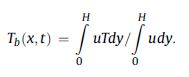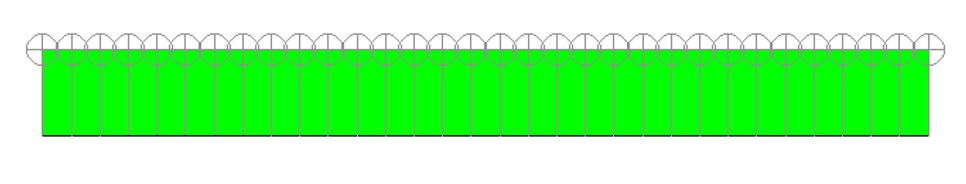## Fluids

•ebrahem
Subscriber

i m calculating bulk temperature or mixed mean temperature of fluid in a 2D domain for that i have to create surfaces

channel height is 3mm and length is 31mm

to create line surfaces i m using scheme file to generate them

(Do ((x -0.004 (+ x 0.0005))) ((> x 0.0271))
(Ti-menu-load-string (format #f "su line-surface x=~a ~a -0.0015 ~a 0.0015" x x x))
)

similar method to create points on wall

at these lines i will calculate Tb and at points on wall i will calculate Tw to use them in Nu calculation

my procedure is simple plot these lines write ascii files with the information of velocity and temperature then by using the matlab i am calculating the bulk temperature to calculate Nusselt number.

i m wondering if someone can guide me that how i can skip matlab and calculate it with fluent only for transient run

as saving data for whole transient run in ASCII format and post process it in matlab is little bit time and storage space consuming

the formula for bulk temperature calculation is similar as discussed in this forum previously i.e. fluent validation for heat transfer.

i hope someone can help me in this regard•Rob
Ansys Employee

Have a look at the monitors: points and volume for Fluent.  Add in Custom Field functions and I think you're there.

•ebrahem
Subscriber

if i may share the procedure here so that u can guide me or correct me if i m doing somehing wrong

i have made the CFF of total temperature*velocity magnitude and named it tu then i make the monitor on the line surface where i take the integral of tu similarly i made the CFF of velocity magnitude and named it u again i make the monitor on line surface and calculate the integral of u

for calculating wall temperature i make the point surfaces and calculate the vertex average of wall temperature on the points.

(Do ((x -0.004 (+ x 0.00001))) ((> x 0.02701))

(Ti-menu-load-string (format #f "su line-surface x=~a ~a -0.0015 ~a 0.0015" x x x))

(Ti-menu-load-string (format #f "s m su set tw~a ~s temperature point-~a () no no yes tw~a 1 yes flow-time" x "Vertex Average" x x))

(Ti-menu-load-string (format #f "s m su set tu~a ~s tu x=~a () no no yes tu~a 1 yes flow-time" x "Integral" x x))

(Ti-menu-load-string (format #f "s m su set u~a ~s u x=~a () no no yes u~a 1 yes flow-time" x "Integral" x x))

)

i dont know how to calculate the Tw -Tb from fluent by adopting above procedure

one more thing fluent become heavy by creating all those surfaces and it also skip some point and line surfaces by showing error

any help is appreciated

below is the image that shows the lines and points for straight channel in low resolution in actual i m making 1000 of lines and points to increase the resolution•Rob
Ansys Employee

Using an average value on a line will reduce the need for points. Then have a look at the various report options and expressions. You may be able to do something clever with parameters and report functions. With 2020R? I think you'll be able to use Expressions for some of this too: can't remember quite what/when stuff is coming.

•ebrahem
Subscriber

if u can share any working example then it will be highly appreciated

•Rob
Ansys Employee

Monitor points & reporting on lines/surfaces is covered in some of the tutorials. You'll find the public tutorials in the Help system, or YouTube videos may well cover reports.

•ebrahem
Subscriber
Please correct me if I am wrong

First I have defined CFF of total temperature × velocity magnitude
Then I defined CFF of velocity magnitude

Next I have made the report function i.e. integral of tu on the interior domain
And similarly integral of u on the interior domain

Next I make the CFF of report definition 1/ report definition 2

And finally make the report definition of new CFF (here I don't know on what surface I have to make it )

Please guide me in the light of above formula have I missed something as in the above mentioned formula we have to find the value as a function of T( x,t )
•Rob
Ansys Employee

You need to work out what you're trying to calculate and check the maths: I'm just going to give you pointers on how to do something.